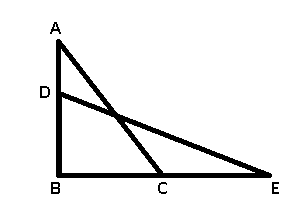Question 76

# A 25 ft long ladder is placed against the wall with its base 7 ft from the wall. The base of the ladder is drawn out so that the top comes down by half the distance that the base is drawn out. This distance is in the range:

SolutionOriginal position of the ladder = AC = $$25$$ ft

Base = BC = $$7$$ ft

=> In $$\triangle$$ ABC, using Pythagoras theorem

=> $$(AB)^2 = (AC)^2 - (BC)^2$$

=> $$(AB)^2 = (25)^2 - (7)^2$$

=> $$AB = \sqrt{625 - 49} = \sqrt{576}$$

=> $$AB = 24$$ ft

After drawing out the base, the new position of ladder = ED = $$25$$ ft

and $$AD = x$$ and $$CE = 2x$$

To find : CE = ?

Solution : In $$\triangle$$ DBE

$$DB = (24 - x)$$ and $$BE = (7 + 2x)$$

=> $$(DB)^2 + (BE)^2 = (ED)^2$$

=> $$(24 - x)^2 + (7 + 2x)^2 = (25)^2$$

=> $$(576 - 48x + x^2) + (49 + 28x + 4x^2) = 625$$

=> $$625 - 20x + 5x^2 = 625$$

=> $$5x^2 = 20x$$

=> $$x = \frac{20}{5} = 4$$

$$\therefore$$ $$CE = 2 \times 4 = 8$$ ft

None of the options include 8 in the interval.